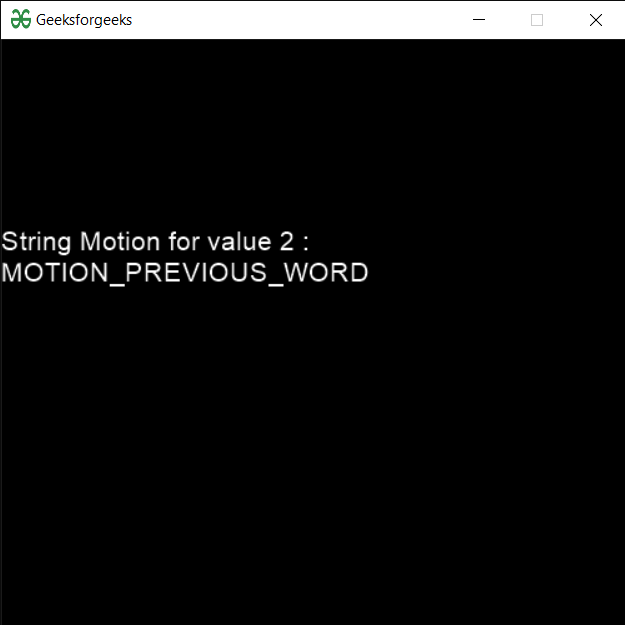Related Articles
PYGLET – Key Motion String
• Last Updated : 23 Sep, 2020

In this article we will see how we can get the key motion string for the modifiers in PYGLET module in python. Pyglet is easy to use but powerful library for developing visually rich GUI applications like games, multimedia etc. A window is a “heavyweight” object occupying operating system resources. Windows may appear as floating regions or can be set to fill an entire screen (fullscreen). Key state handler class is a simple handler that tracks the state of keys on the keyboard. If a key is pressed then this handler holds a True value for it. Motion string is string describing a text motion.

We can create a window with the help of command given below

```# creating a window
window = pyglet.window.Window(width, height, title)

```

In order to create window we use `motion_string` method with the pyglet.window.key

Syntax : key.motion_string(motion)

Argument : It takes integer as argument

Return : It returns string

Below is the implementation

 `# importing pyglet module ``import` `pyglet ``import` `pyglet.window.key as key``   ` `# width of window ``width ``=` `500``   ` `# height of window ``height ``=` `500``   ` `# caption i.e title of the window ``title ``=` `"Geeksforgeeks"``   ` `# creating a window ``window ``=` `pyglet.window.Window(width, height, title) ``   ` `# text  ``text ``=` `"Welcome to GeeksforGeeks Have a nice day"``   ` `# batch object ``batch ``=` `pyglet.graphics.Batch() ``   ` `# creating document ``document ``=` `pyglet.text.document.FormattedDocument(text) ``   ` `# setting style to the document ``document.set_style(``0``, ``len``(document.text), ``dict``( ``                         ``font_name ``=``'Arial'``, font_size ``=` `16``,  ``                         ``color ``=``(``255``, ``255``, ``255``, ``255``))) ``   ` `# creating a incremental text layout ``layout ``=` `pyglet.text.layout.IncrementalTextLayout( ``               ``document, ``400``, ``350``, batch ``=` `batch) `` ` `# making layout to display multiline``layout.multiline ``=` `True``   ` `# creating a caret ``caret ``=` `pyglet.text.caret.Caret(layout, color ``=``(``255``, ``255``, ``255``)) ``   ` `# caret to winow push handlers ``window.push_handlers(caret) ``   ` `# setting caret style ``caret.set_style(``dict``(font_name ``=``"Arial"``)) ``   ` `   ` `# on draw event ``@window``.event ``def` `on_draw(): ``       ` `    ``# clear the window ``    ``window.clear() ``       ` `    ``# draw the batch ``    ``batch.draw() ``       ` `    ``# caret to winow push handlers ``    ``window.push_handlers(caret) ``     ` `     ` ` ` `# creating a key state handler``key_handler ``=` `pyglet.window.key.KeyStateHandler()``       ` `# key press event     ``@window``.event ``def` `on_key_press(symbol, modifier): ``   ` `    ``# key "C" get press ``    ``if` `symbol ``=``=` `key.C: ``         ` `        ``# printng the message``        ``print``(``"Key : C is pressed"``)``         ` `# image for icon ``img ``=` `image ``=` `pyglet.resource.image(``"gfg.png"``) `` ` `# getting motion string``value ``=` `key.motion_string(``2``)`` ` `# creating text from the value``text ``=` `"String Motion for value 2 : "` `+` `str``(value)`` ` `# setting this text to the document``document.text ``=` `text``   ` `# setting image as icon ``window.set_icon(img) ``    ` `# start running the application ``pyglet.app.run() `

Output :Attention geek! Strengthen your foundations with the Python Programming Foundation Course and learn the basics.

To begin with, your interview preparations Enhance your Data Structures concepts with the Python DS Course. And to begin with your Machine Learning Journey, join the Machine Learning – Basic Level Course

My Personal Notes arrow_drop_up